# Traffic Lights

Have you ever wondered what the probability is of making it through a sequence of traffic lights without stopping? Me neither. But some people do so let’s try and solve that problem. It’s something that appears in everyday life for example my walk from home to DroneDeploy taken me through 16 traffic lights.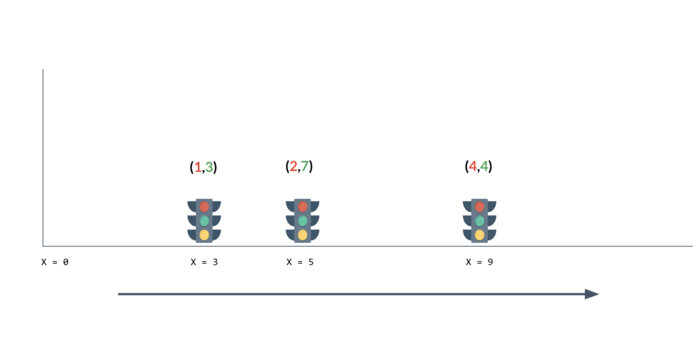Let’s say we begin at position `X=0` at time `T` in seconds and start walking as `1` meter per second right. Let’s say `T` is uniformly randomly distributed in the range `[0, 1e100]` to account for all manner of late starts. Then let’s say there are `N` traffic lines as positions `X1, X2, … ,XN` for each of these they are red for `Ri` seconds, then green for `Gi` seconds after which they repeat their cycles. At time `T= 0` all traffic lights have just turned red. So givens the lists or `X`, `R` and `G` let’s try and compute the probability that we hit each of the red lights and thus the probability that we make it all the way through. The images above shows `4` traffic lights with periods `8`, `22`, `6` and `10`. Let’s also say `R + G <= 100`.

Our first attempt could be to samples start times `T` and for each sample compute which traffic light we stop at (if any) and report the probabilities.

``````Light = namedtuple('Light', ['x', 'r', 'g'])

lights = [
Light(3, 1, 3),
Light(5, 2, 7),
Light(9, 4, 4),
]

def simulate(t, lights):
for index, light in enumerate(lights):
at = (light.x + t) % (light.r + light.g)
if at < light.r:
return index
return len(lights)

def solution(lights):

N = len(lights)
SAMPLES = 1000000
P = 1
for light in lights:
P = lcm(P, light.r+light.g)

ret =  * (N + 1)

for _ in range(SAMPLES):
T = np.random.uniform(0, 1e100)
i = simulate(T, lights)
ret[i] += 1

count = sum(ret)
return [ i / count for i in ret]
``````

Here’s what a few samples might look like. The solution is easy to code but it’s really slow and unlikely to converge to something useful in a reasonable amount of time.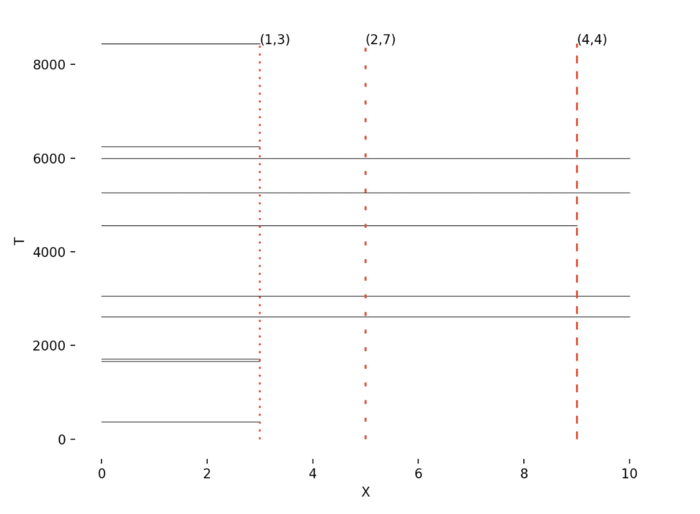Let’s hunt for a more effective solution. Let’s look at the periods of the traffic lights `Pi = Ri+Gi`. Given that the lights start having just turned red the system will start repeating at some point in time. Computing the lowest common multiple of the periods `Pi` gives the amount of times for the system to repeat. So a better solution would be instead of trying random start time to try each start time in the range `[ 0 … LCM([P1, P2, ... , PN]) ]` and then compute the probability.

``````def gcd(a, b):
if b > 0:
return gcd(b, a%b)
else:
return a

def lcm(a, b):
return a * b // gcd(a, b)

def solution(lights):

N = len(lights)
P = 1
for light in lights:
P = lcm(P, light.r+light.g)

ret =  * (N + 1)

for T in range(P):
i = simulate(T, lights)
ret[i] += 1

count = sum(ret)
return [ i / count for i in ret]
``````

This works and gives us a `O ( LCM([P1, P2, ... , PN]) )` solution. Here’s what it look like for the same system: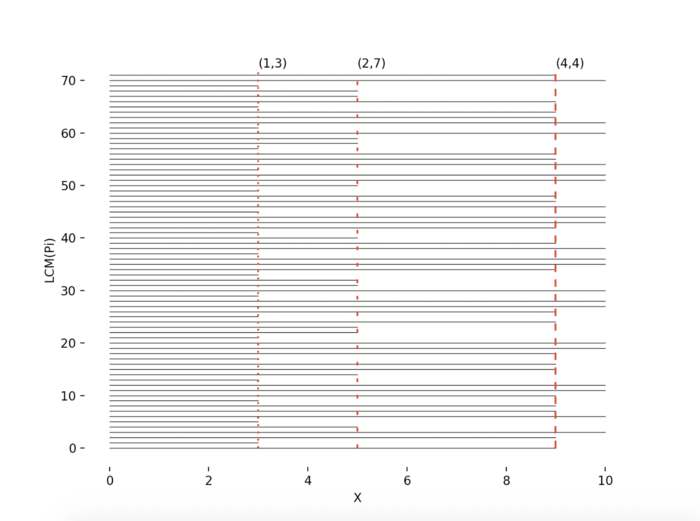On the y-axis we have all possible start times modulo `LCM([P1, P2, ... , PN])`, as we don’t need to consider any others. The arrows represent which traffic like we would hit starting at that time. The final probabilities are correct so let’s look at the worst case of having traffic lights of all the periods between `1` and `100`. What is the LCM of the numbers `1 .. 100`. Uh oh, it’s `69,720,375,229,712,477,164,533,808,935,312,303,556,800`. That’s not going to work. Can we do better?

Let’s look at the system of period `3` and `5` traffic lights. The system will repeat with a period of `LCM(3, 5) = 15`. But in addition the periods are co-prime as they share no prime factors `GCD(3, 5) = 1`. Which means knowing what state the period `3` traffic light is in tells us nothing about what state the period `5` traffic light is in. Essentially the probabilities are independent and the probability of making it through both lights is just the probability of making it through the first light multiples by the probability of making it through the second light, `G1 / (R1+G1) * G2 / (R2+G2)`. Maybe `R2G2` will feature in the new Star Wars. Anyway, this is true for a set of traffics lights with co-prime periods like. This yields a nice `O(N)` solution with the caveat that the periods must be co-prime.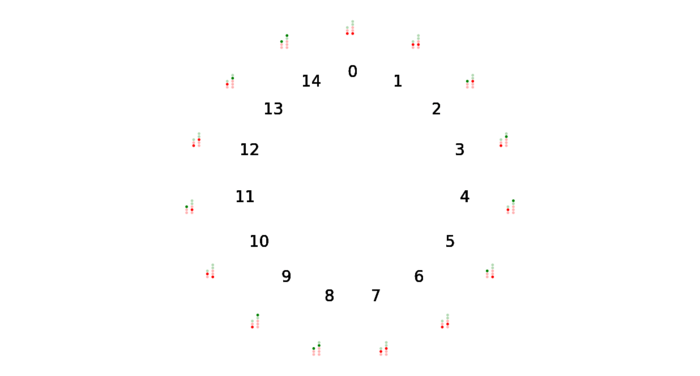Here’s what things look like if we add another light of period `2` to the system.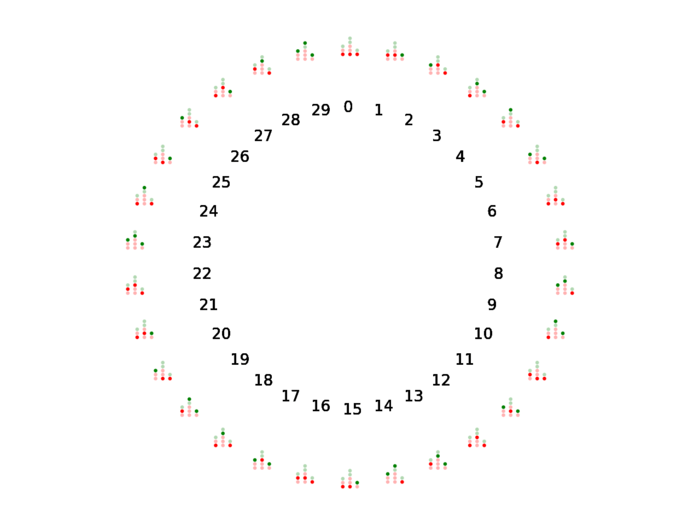Now what happens if we introduce a period `6` light into this set. In this case `6` is a multiple of the existing period.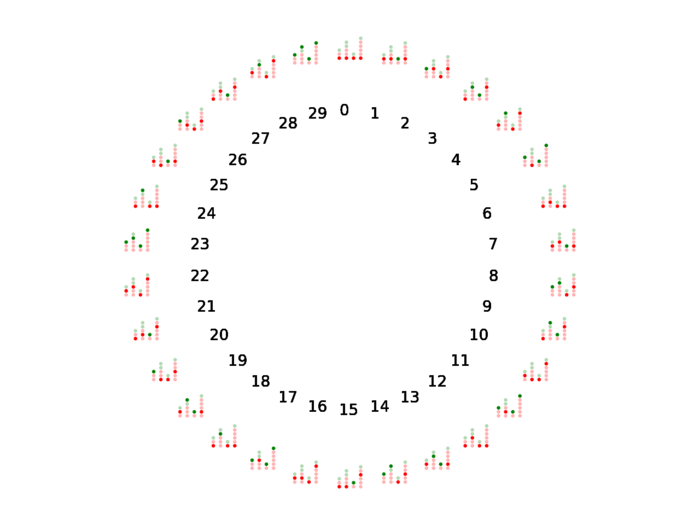This is the same as repeating the existing period `2` and `3` traffic lights. So it’s the same as this system.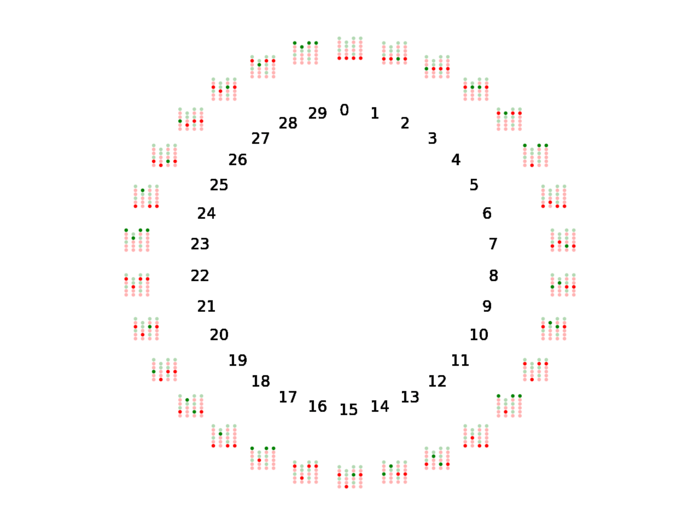Thus for a set of periods which are either co-prime or multiples of each other we can solve the system in `O(N * P)` where `P` in the largest period, by considering each time `0 … P-1` and keeping track of which times modulo that period would hit a red light. We can do this by computing for each light which light to it’s left have periods which are multiples of itself, or where current light is a multiple of a previous one and instead use the period of that light.

Great! But what about these two lights, `8` and `12`. They are neither co-prime nor integer multiples of each other as they share a factor `GCD(8, 12) = 4`. Let’s pick another number `Z` and consider start times modulo `Z`: `{ T+Z, T+2Z, …. }`. If we only consider these times a traffic light with period `P` will still be periodic but will have a period of `P / GCD(Z, P)`. For the example let’s pick `Z` as `4` and create a system of reduced periods `2` and `3`, (originally `8` and `12`). These are co-prime so we can use our initial algorithm. The problem is now a matter of finding the suitable value `Z` that for a set of periods transforms them to a set of reduced periods where each is either co-prime or a multiple of another period. Here some code to compute one of those numbers experimentally:

``````def findZ():

nums = list(range(100))

again = True
factors = []

while again:
again = False
for i in range(len(nums)):
for j in range(i+1, len(nums)):

if nums[i] % nums[j] == 0 or nums[j] % nums[i] == 0:
continue

z = gcd(nums[i], nums[j])
if z != 1:
for k in range(len(nums)):
if nums[k] % z == 0:
nums[k] = nums[k] // z

factors.append(z)
again = True

return factors
``````

The result is the factors `[2, 2, 2, 2, 3, 3, 5, 7]` the product of which is `2520`. That’s the key we’ve been looking for. This leads us to the following solution which runs in a very manageable `O(N * X * P)`.

``````def solution(lights):

Z = 2520
N = len(lights)

fn = [ ]
filters = []
for i, light in enumerate(lights):
period = (light.r+light.g) // gcd(Z, light.r+light.g)
for j in range( len(filters) ):

if period % len(filters[j]) == 0:
filters[j] = [-1] * period

if len(filters[j]) % period == 0:
fn.append(j)
break
else:
fn.append( len(filters) )
filters.append( [-1] * period )

ret =  * (N + 1)

for base in range(Z):
cur = 1.0 / Z
for i, light in enumerate(lights):
filter = filters[fn[i]]
total = 0
hits_red = 0
t = Z + base + light.x
for j in range(len(filter)):
if filter[j] < base:
total += 1
if t % (light.r + light.g) < light.r:
filter[j] = base
hits_red += 1
t += Z
entering = cur
if (total):
cur *= (total - hits_red) / total
ret[i] += entering - cur
ret[N] += cur

return ret
``````

It’s really cool to see a seemly intractable problem dissolve in the presence of a seemingly magic number that disconnects all the primes. So what’s the probability of making is through a sequence of traffic lights? Wonder no more. This question is based on ICPC World Finals 2019 Problem K.